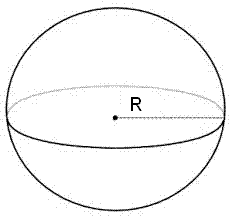# Sphere Calculator

Calculator will calculate the Volume of Sphere and Curved Surface Area(CSA) of Sphere for given radius values:Volume of Sphere: [ (4/3)πr³ ] Enter the Radius = Volume of Sphere =Curved Surface Area(CSA) of Sphere: [ 4πr² ] Enter the Radius = Curved Surface Area(CSA) of Sphere =